HOME COURSES PREVIEW REVIEW ABOUT CONTACTTOLL-FREE INFO & ORDERING: M-F: 9am-5pm (PST): (877) RAPID-10

 Quick Search: Keywords:

 Rapid Learning Member Area:Note: If you are a legacy user of chemistry24 members, please request a new login access to the premium server with your full name and old login email via vip@rapidlearningcenter.com

 Rapid Courses Catalog : Physics in 24 Hours Chemistry in 24 Hours Biology in 24 Hours Mathematics in 24 Hours

Weekly Physics Tips:
Want to become a top gun in your class? How about study less yet score high? Sign up this Physics Survival Weekly to learn how. Designed specifically for students who are taking physics, this free newsletter will show you how to survive and excel in class! Weekly topics include:
• How to Study Physics Effectively
• How to Read Physics Textbooks Easily
• How to Solve Physics Problems Systematically
• How to Score High on Physics Exams Strategically
• How to Master Physics Rapidly
Each week, you will receive study tips on the topics above and visual tutorial or study template to enhance your physics learning. Enter your name and email below to subscribe free:

 Physics Study Lounge These study sheets are for quick review on the subjects. Refer to our rapid courses for comprehensive review.     - Basic Skills in Physics     - Basic Math for Physics     - How to Solve Physics Problems     - Newton's Laws Study Guide     - ElectroMagnetism Quick Review     - Atomic Physics At-A-Glance     - Core Concepts in Nuclear Physics     - Special Relativity Overview

 Other Related Sites
 Note: For course links to launch, disable popup blockers or hold the ctrl key while clicking the link.Home » College Calculus

Fundamental Differentiation Techniques

 Topic Review on "Title": The Constant Rule of Derivatives: If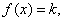and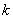is a real number constant then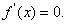The Power Rule of Derivatives: If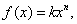and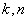are real numbers then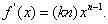The sum and difference rule of derivatives: If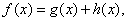then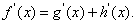If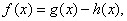then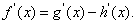The product rule of derivatives: If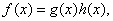then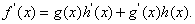The quotient rule of derivatives: If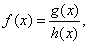then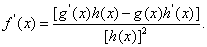The Chain Rule of Derivatives: If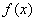is a composition of two differentiable functions,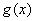and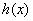such that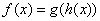then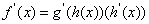then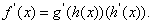Rapid Study Kit for "Title":
 Flash Movie Flash Game Flash Card Core Concept Tutorial Problem Solving Drill Review Cheat Sheet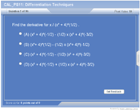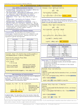"Title" Tutorial Summary : This tutorial presents the techniques for quickly finding the derivative of monomials. Examples are introduced to show the techniques for quickly finding the derivative of polynomials. Theorems are introduced in this tutorial containing the most basic principles used in finding derivatives of functions. Next, this tutorial introduces the techniques for quickly finding the derivative of trigonometric functions. Also, the way to find the derivative of composite functions is mentioned in this tutorial.

 Tutorial Features: Specific Tutorial Features: Step by step example problems showing how you calculate the derivative of monomials, the derivative of polynomials and the derivative of composite functions. The Chain Rule is introduced with the aid of example problems and its graphical representation. Series Features: Concept map showing inter-connections of new concepts in this tutorial and those previously introduced. Definition slides introduce terms as they are needed. Visual representation of concepts Animated examples—worked out step by step A concise summary is given at the conclusion of the tutorial.

 "Title" Topic List: `Derivatives of monomials Derivative techniques Power rule for a fractionDerivatives of polynomials Sum and difference rule for derivatives Product rule for derivatives Quotient rule for derivativesDerivatives of trigonometric functionsThe Chain Rule for derivatives`

See all 24 lessons in college calculus, including concept tutorials, problem drills and cheat sheets:
Teach Yourself College Calculus Visually in 24 Hours